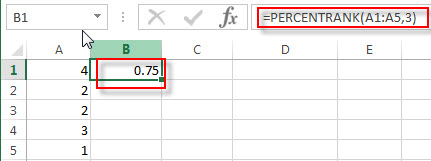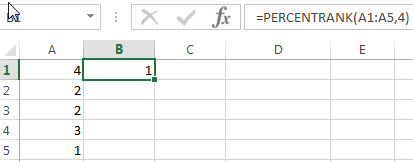## Excel PERCENTRANK Function

This post will guide you how to use the PERCENTRANK function with syntax and examples in Microsoft excel.

### Description

The Excel PERCENTRANK function returns the rank of a value in a set of values as a percentage of the set. So you can use the PERCENTRANK function to calculate the relative position of a value in a set of values as a percentage in Excel. This function has been replaced by the PERCENTRANK .INC function in Excel 2010.

The PERCENTRANK function is a build-in function in Microsoft Excel and it is categorized as a Statistical Function.

The PERCENTRANK function is available in Excel 2016, Excel 2013, Excel 2010, Excel 2007, Excel 2003, Excel XP, Excel 2000, Excel 2011 for Mac.

### Syntax

The syntax of the PERCENTRANK function is as below:

`= PERCENTRANK (array,x,[significance])`

Where the PERCENTRANK function arguments are:

• Array – This is a required argument.  A range or array or cell reference from which you want to get the rank of a specific value.
• X – This is a required argument. The value that you want to find the rank for.
• Significance -This is an optional argument.  A value that identifies the number of significant digits for the returned percentage value. If omitted, PERCENTRANK uses three digits (0.xxx).

Note:

• If the array argument is empty, the PERCENTRANK function will return the #VALUE! Error.
• If the significance argument is small than 1, the PERCENTRANK function will return the #NUM! Error.
• If x does not match one of the values in the array, the PERCENTRANK function will interpolate to find the percentage rank.

### Excel PERCENTRANK Function Examples

The below examples will show you how to use Excel PERCENTRANK Function to get the rank of a value in a data set as a percentage of the data set.

Example 1:  to get the percent rank of 3 in the range A1:A5, using the following formula:

`= PERCENTRANK (A1:A5)`Because there are 3 values are less than 3, and 1 value is greater than 3, so the percent rank of 3 in the range A1:A5 is: 3/(3+1)=0.75

Example 2:  to get the percent rank of 4 in the range A1:A5, using the following formula:

`= PERCENTRANK (A1:A5)`Because there are 4 values are less than 4, and 0 value is greater than 4, so the percent rank of 4 in the range A1:A5 is: 4/(4+0)=0.75